# Properties of Triangle:

We need to study about triangle and its properties from class 7 and till class 9 we know much about a triangle and its properties. There are some properties of triangle which tells whether there is its existence or not.

## Let’s summarize all the properties of triangle beneficial to us:

1. ### Angle sum property of a triangle for class 7:

• The sum of all interior angle of a triangle is equal to 180⁰.

∠A + ∠B + ∠C = 180⁰

###2. Exterior angle properties of triangle: The measure of any exterior angle of a triangle is equal to sum of opposite two interior angles.The exterior angle ∠4 = ∠1 + ∠2

3. Equiangular property:

• The angle opposite to equal sides of a triangle (especially isosceles triangle) are equal in measure.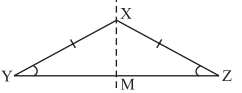• All angles of an equilateral triangle are equal and has a measure of 60⁰ each.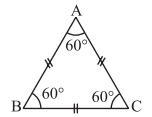1. ### Equal side Property:

• Sides opposite to equal angles of a triangle (especially isosceles triangle) are equal in measure.• All sides of an equilateral triangle are equal.1. ### Right triangle property:

• In a right triangle, the square of the hypotenuse is equal to the sum of the squares of the other two sides (Pythagoras Theorem).

a2 + b2 = c2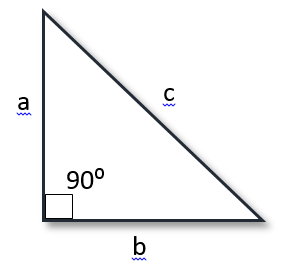1. ### The central angle of equilateral triangle:

• The measure of every central angle opposite to each sides has a measure of $$\frac{360⁰}{3}$$ = 120⁰.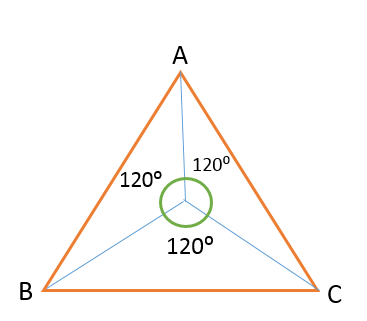1. ### Inequality in a triangle:

• If two sides of a triangle are of different length, then the angle opposite to the longer side is larger (or greater).
1. ### Longer side property:

• In any triangle, the side opposite to larger angle is greater in measure.
1. ### Important inequality properties of triangle for class 9:

• The sum of any two sides of a triangle is always greater than the third side.
1. ### Congruent Triangle property for class 9:

• If two triangles are congruent then all the sides and angles of one triangle is exactly equal to the corresponding sides and angles of the other triangle.
1. ### Similar triangle property:

• If two triangles are similar than every angle of one triangle is equal to the corresponding angle of the other triangle and when side is compared all sides must be in the same proportion to the sides of other triangle.
1. ### Basic proportionality theorem or Thales theorem for class 10:

• The ratio of any two corresponding sides in two equiangular triangles when compared, is always the same.
1. ### Parallel lines in a triangle property:

• If a line is drawn parallel to one side of a triangle to intersect the other two sides at distinct points, then the other two sides are divided in the same ratio.

i.e. $$\frac{AD}{DB}$$ = $$\frac{AE}{EC}$$1. ### Converse Of the above is also true:

• If a line divides any two sides of a triangle in the same ratio, then the line is parallel to the third side.

i.e. $$\frac{AD}{DB}$$ = $$\frac{AE}{EC}$$1. ### Area property of similar triangles:

• The ratio of the areas of two similar triangles is equal to the square of the ratio of their corresponding sides.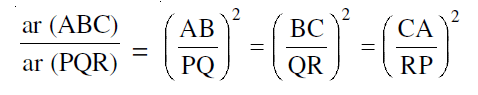• To get further information about properties of triangle please visit connect study

1.Prachi Rathore March 14, 2020